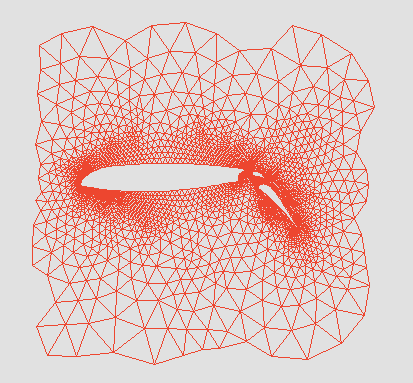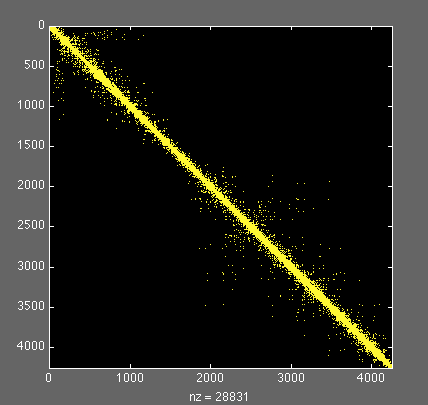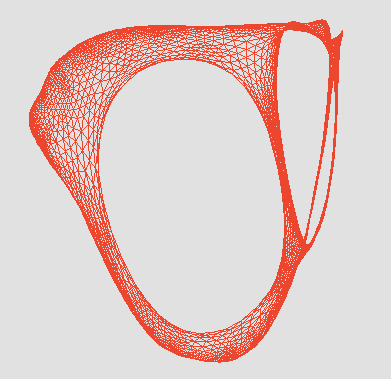## Sparse Matrix Algorithms

### Computer Science 219, Spring 2018

Schedule, Readings, and Homework nvsbl Assignments nvsbl Discussion Forum nvsbl Matlab nvsbl References nvsbl Final Projects nvsblMon/Wed 9:00-10:50
Phelps 3526
John R. Gilbert

Sparse matrices are a basic tool of computational science and engineering. They show up in applications ranging from models of the physical world to web search and graph clustering. Using them efficiently involves techniques from linear algebra, graph algorithms, and computer architecture.

Sparse matrix algorithms are fascinating (to me at least :) because they combine two languages that are often quite different, those of numerical computation and of graph theory. One result is that nobody knows it all -- there is always something new to be learned by trying to speak the language you're not expert in.

Most of the course will concern methods for solving large, sparse systems of linear equations. We will study direct methods, which are based on Gaussian elimination and use tools from graph theory and discrete data structures; iterative methods, which treat the matrix as a black-box operator and use eigenvalues and eigenvectors to analyze convergence; and modern preconditioned methods, which combine the discrete structure of direct methods, the numerical structure of iterative methods, and the specifics of the problem domain. We'll also study some methods for finding eigenvalues and eigenvectors of large, sparse matrices.

The prerequisites are some knowledge of linear algebra (Gaussian elimination, eigenvalues and eigenvectors) and analysis of algorithms. I expect to have students with a variety of different backgrounds; if you have an application from a scientific or engineering field that includes solving a system of linear equations I encourage you to talk to me about the course.

Students will do homework assignments and a term project. The term project can be either an application to a real computational science problem, an algorithms implementation experiment, or a theoretical or survey paper.

Approximate course outline:

• The basics:
• Graphs and matrices
• Data structures for sparse matrix manipulation
• Linear solvers and their complexity
• Sparse Gaussian elimination:
• Cholesky factorization
• Elimination trees, symbolic factorization, structure prediction
• Orderings for low fill
• Symmetric indefinite and nonsymmetric systems
• Krylov-subspace iterations:
• Convergence analysis of CG
• GMRES, BiCGSTAB, and friends
• Preconditioned methods:
• Incomplete factorization
• Sparse approximate inverses
• Support theory
• Multigrid
• Sparse eigenvalue/eigenvector algorithms